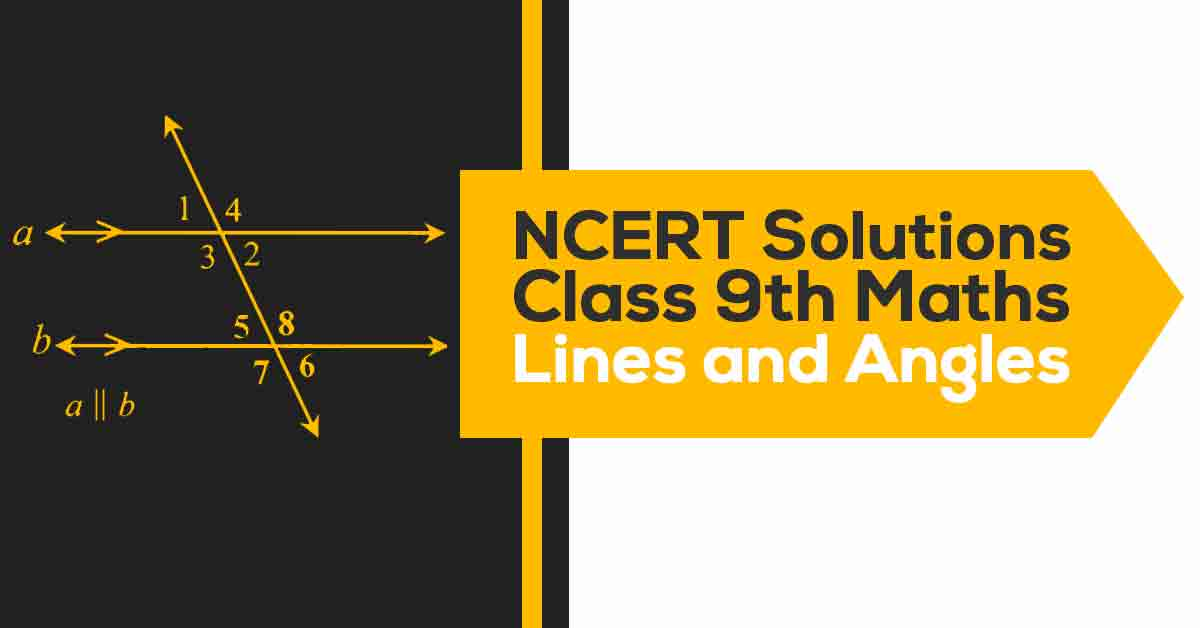NCERT Solutions for Class 9 Maths Chapter 6 | Vidyakul
×

# NCERT Solutions For Class 9 Maths Chapter 6

## NCERT Solutions For Class 9 Maths Chapter 6 Lines and Angles  - PDF DownloadStudents can take help from NCERT Solutions for Class 9 Maths Chapter 6 Lines and Angles to study for their examinations. In this chapter, you will study the properties of the angles formed when two lines intersect each other, and also the properties of the angles formed when a line intersects two or more parallel lines at distinct points. Further, you will use these properties to prove some statements using deductive reasoning.

These CBSE NCERT Solutions for Class 9 Maths Chapter 6 Lines and Angles will help you to practice all the topics in the chapter with ease and score maximum marks in the examination.

What is a straight Line?

A straight line is defined as the line which had neither starting nor endpoint and it is of infinite length.

What are angles?

Angles are of two types:

1. Acute angle: The angle between 0degrees and 90 degrees is an acute angle.

2. Obtuse angle: The angle between 90 degrees and 180 degrees is an obtuse angle.